### Home > MC2 > Chapter 9 > Lesson 9.1.3 > Problem9-29

9-29.
1. Triangle ABC is similar to triangle DEF. Homework Help ✎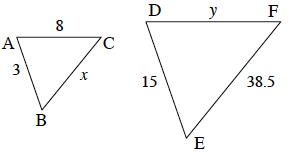1. Find the scale factor from triangle ABC to triangle DEF.

2. Find x.

3. Find y.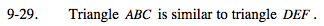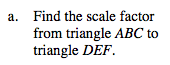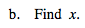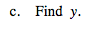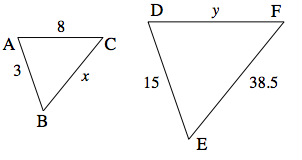Find the scale factor between two given similar sides for the two shapes.

The scale factor is 5.

Divide by the scale factor.

$x=\frac{38.5}{5}$

Multiply the matching side by the scale factor.